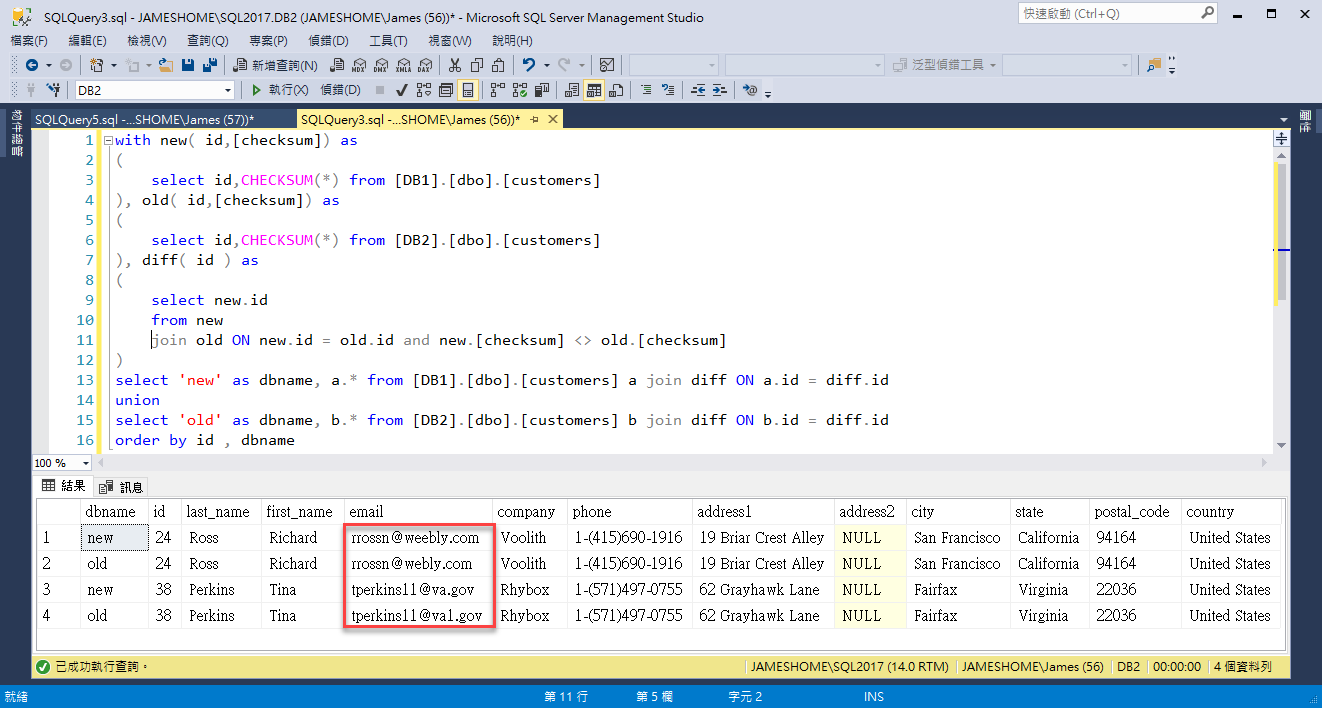### [SQL]利用 checksum 來簡化資料表比對問題

``````with new( id,[checksum]) as
(
select id,CHECKSUM(*) from [DB1].[dbo].[customers]
), old( id,[checksum]) as
(
select id,CHECKSUM(*) from [DB2].[dbo].[customers]
), diff( id ) as
(
select new.id
from new
join old ON new.id = old.id and new.[checksum] <> old.[checksum]
)
select 'new' as dbname, a.* from [DB1].[dbo].[customers] a join diff ON a.id = diff.id
union
select 'old' as dbname, b.* from [DB2].[dbo].[customers] b join diff ON b.id = diff.id
order by id , dbname````````````with new( tablename, checksum )  as
(
select 'customers' as tablename,CHECKSUM_AGG( CHECKSUM(*)) as checksum from [DB1].[dbo].[customers]
union
select 'employees' as tablename,CHECKSUM_AGG( CHECKSUM(*)) as checksum from [DB1].[dbo].[employees]
union
select 'orders' as tablename,CHECKSUM_AGG( CHECKSUM(*)) as checksum from [DB1].[dbo].[orders]
union
select 'order_details' as tablename,CHECKSUM_AGG( CHECKSUM(*)) as checksum from [DB1].[dbo].[order_details]
union
select 'products ' as tablename,CHECKSUM_AGG( CHECKSUM(*)) as checksum from [DB1].[dbo].[products]
), old( tablename, checksum )  as
(
select 'customers' as tablename,CHECKSUM_AGG( CHECKSUM(*)) as checksum from [DB2].[dbo].[customers]
union
select 'employees' as tablename,CHECKSUM_AGG( CHECKSUM(*)) as checksum from [DB2].[dbo].[employees]
union
select 'orders' as tablename,CHECKSUM_AGG( CHECKSUM(*)) as checksum from [DB2].[dbo].[orders]
union
select 'order_details' as tablename,CHECKSUM_AGG( CHECKSUM(*)) as checksum from [DB2].[dbo].[order_details]
union
select 'products ' as tablename,CHECKSUM_AGG( CHECKSUM(*)) as checksum from [DB2].[dbo].[products]
)
select new.* from new join old on new.tablename=old.tablename and new.checksum<>old.checksum``````